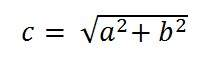# Pixels, Dots and Lines Per Inch

In the previous tutorial of spatial resolution , we discussed the brief introduction of PPI, DPI, LPI. Now we are formally going to discuss all of them.

## Pixels per inch

Pixel density or Pixels per inch is a measure of spatial resolution for different devices that includes tablets, mobile phones.

The higher is the PPI, the higher is the quality. In order to more understand it, that how it calculated. Lets calculate the PPI of a mobile phone.

### Calculating pixels per inch (PPI) of Samsung galaxy S4:The Samsung galaxy s4 has PPI or pixel density of 441. But how does it is calculated?

First of all we will Pythagoras theorem to calculate the diagonal resolution in pixels.

It can be given as:Where a and b are the height and width resolutions in pixel and c is the diagonal resolution in pixels.

For Samsung galaxy s4, it is 1080 x 1920 pixels.

So putting those values in the equation gives the result

C = 2202.90717

Now we will calculate PPI

PPI = c / diagonal size in inches

The diagonal size in inches of Samsung galaxy s4 is 5.0 inches , which can be confirmed from anywhere.

PPI = 2202.90717/5.0

PPI = 440.58

PPI = 441 (approx)

That means that the pixel density of Samsung galaxy s4 is 441 PPI.

## Dots per inch.

The dpi is often relate to PPI, whereas there is a difference between these two. DPI or dots per inch is a measure of spatial resolution of printers. In case of printers, dpi means that how many dots of ink are printed per inch when an image get printed out from the printer.

Remember, it is not necessary that each Pixel per inch is printed by one dot per inch. There may be many dots per inch used for printing one pixel. The reason behind this that most of the color printers uses CMYK model. The colors are limited. Printer has to choose from these colors to make the color of the pixel whereas within pc, you have hundreds of thousands of colors.

The higher is the dpi of the printer, the higher is the quality of the printed document or image on paper.

Usually some of the laser printers have dpi of 300 and some have 600 or more.

## Lines per inch

When dpi refers to dots per inch, liner per inch refers to lines of dots per inch. The resolution of halftone screen is measured in lines per inch.

The following table shows some of the lines per inch capacity of the printers.

Printer LPI
Screen printing 45-65 lpi
Laser printer (300 dpi) 65 lpi
Laser printer (600 dpi) 85-105 lpi
Offset Press (newsprint paper) 85 lpi
Offset Press (coated paper) 85-185 lpi